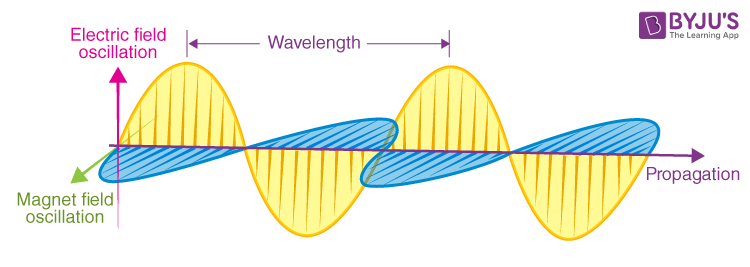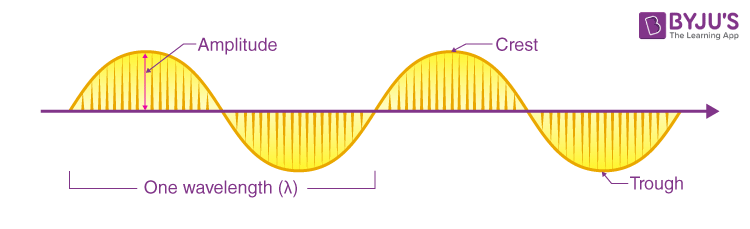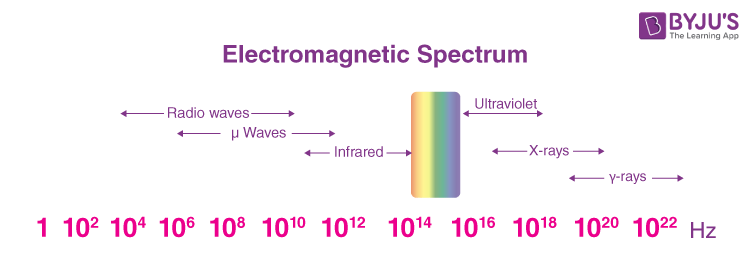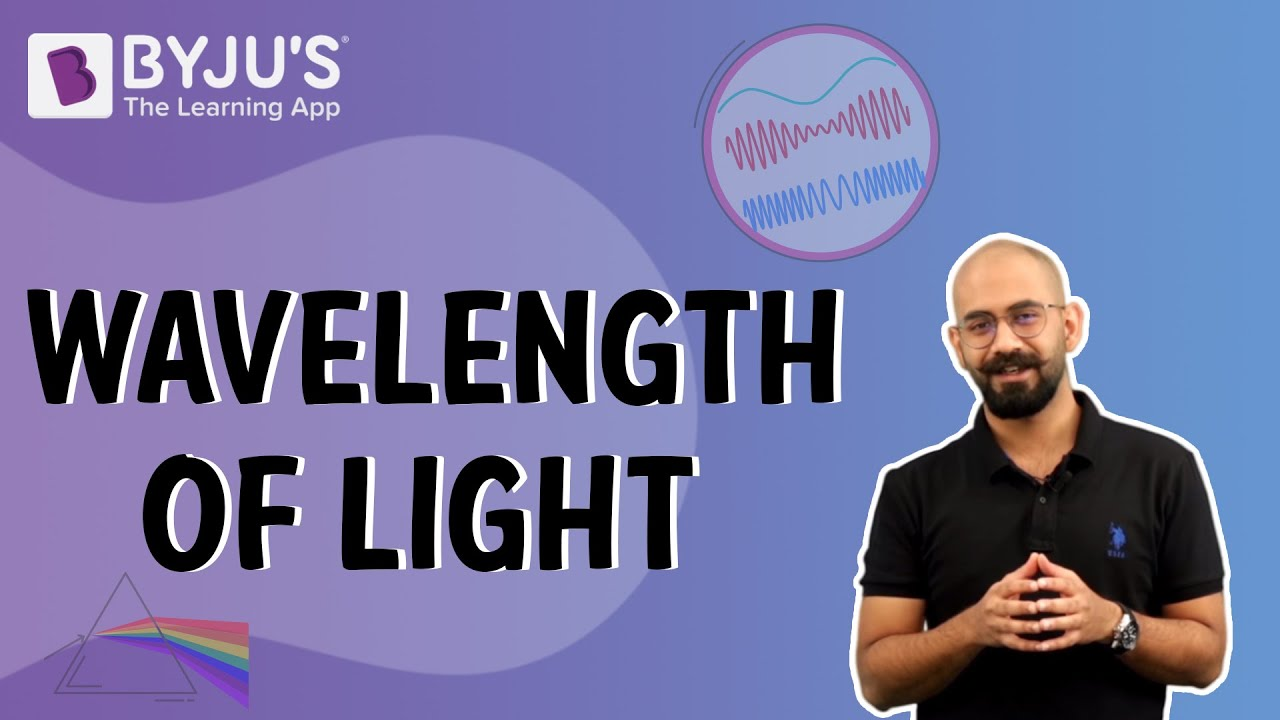Electromagnetic radiation, or EM radiation, is a fundamental form of energy that surrounds us everywhere, from the microwaves that heat our food to the radio waves that bring us music and news. In this article, we will explore electromagnetic radiation, its nature, theory, properties, and the wide range of forms it takes.Electromagnetic radiation can be defined as a form of energy that is generated when electrically charged particles move through matter or a vacuum. Alternatively, it can also be produced by oscillating magnetic and electric disturbances.Let’s delve deeper into the key aspects of electromagnetic radiation:

• Energy from Charged Particles: Electromagnetic radiation is generated when electrically charged particles, such as electrons, move through a medium or empty space. These particles carry energy as they travel, creating a wave-like pattern of electric and magnetic fields.
• Electric and Magnetic Fields:Electromagnetic radiation consists of electric and magnetic fields. These fields are intimately connected and perpendicular to each other, with a 90-degree angle. As the combined waves propagate through space, they move perpendicular to the electric and magnetic oscillations occurring during the disturbance.
• Diverse Forms of Electromagnetic Radiation: Electromagnetic radiation encompasses a vast spectrum of energy, ranging from low-energy radio waves to high-energy gamma rays. Some common forms of electromagnetic radiation include microwaves, television waves, radio waves, infrared radiation, visible light, ultraviolet radiation, X-rays, and gamma rays. Each form has different wavelengths and energy levels, resulting in unique properties and applications.
• Transverse Waves: Electromagnetic radiation travels as transverse waves, meaning that the oscillations occur perpendicular to the direction of wave propagation. This characteristic allows electromagnetic radiation to traverse space, enabling it to propagate through a vacuum, unlike other types of waves.• Photons: Electromagnetic radiation is emitted as discrete packets of energy called photons. These photons carry light energy and travel at the constant speed of light.
• Quantized Harmonic Waves: Electromagnetic radiation can be described as quantized harmonic waves. These waves exhibit specific frequencies and energy levels.
• Categorization by Wavelength: Electromagnetic radiation is grouped into different categories based on its wavelength within the electromagnetic spectrum. Each category has distinct properties and applications.
• Perpendicular Electric and Magnetic Fields: Electromagnetic waves consist of perpendicular electric and magnetic fields. These fields oscillate in directions that are perpendicular to each other and to the direction of wave propagation.
• Wavelength, Amplitude, and Frequency: Electromagnetic radiation exhibits properties such as wavelength, amplitude, and frequency. Wavelength (λ) represents the distance between two adjacent points in phase, while frequency determines the number of wave cycles per second. Amplitude refers to the maximum displacement of the wave from its equilibrium position.
• Travel through Empty Space: Unlike other types of waves, electromagnetic radiation can travel through empty space. It does not require a medium such as a solid, liquid, or gas for propagation.
• Constant Speed of Light: The speed of light in a vacuum, denoted as “c,” is a fundamental constant of approximately 2.99792458 x 108 metres per second. This speed remains constant for all forms of electromagnetic radiation.

Understanding the properties of electromagnetic radiation allows us to comprehend its behaviour, interactions with matter, and various applications across scientific disciplines and everyday life.#### Gamma-rays

Gamma rays have the highest frequency and photon energy in the electromagnetic spectrum. They have extremely short wavelengths and are associated with nuclear processes and high-energy particle interactions.

#### X-rays

X-rays have frequencies and photon energies slightly lower than gamma rays. They are widely used in medical imaging, security screening, and industrial applications due to their ability to penetrate materials and reveal internal structures.

#### Ultraviolet (UV) Rays

Ultraviolet rays have higher frequencies and photon energies than visible light. They are emitted by the Sun and play a crucial role in biological processes, such as vitamin D synthesis and triggering chemical reactions in the atmosphere.

#### Visible Light:

Visible light is the portion of the electromagnetic spectrum visible to the human eye. Visible light is essential for vision. It has a range of frequencies and photon energies that allow us to perceive different colours.

#### Infrared (IR) Rays

Infrared rays have lower frequencies and photon energies than visible light. They are associated with thermal radiation and are commonly used in night vision, remote sensing, and infrared spectroscopy applications.

Radio waves have the lowest frequencies and photon energies in the electromagnetic spectrum. They are used for communication, broadcasting, and radar systems. Different portions of the radio wave spectrum are allocated for various applications, including AM and FM radio, television, and mobile communication.

#### Microwaves

Microwaves have slightly higher frequencies and photon energies than radio waves. They are commonly used in microwave ovens, satellite communication, and wireless technologies.

Understanding the electromagnetic spectrum allows us to explore the diverse range of electromagnetic radiation and its applications.

## Discover the key differences between infrared, visible, and ultraviolet radiation in this video tutorial!Understanding the characteristics of electromagnetic waves is crucial to comprehending the behaviour and applications of electromagnetic waves. Let’s explore these characteristics in more detail:

### Wavelength

Wavelength (λ) represents the distance between successive crests or troughs of a wave. It is the length of one complete cycle of oscillation. Wavelength and frequency (ν) are related by the equation c = λν, where ‘c’ is the speed of light. Shorter wavelengths correspond to higher frequencies and higher energy levels.

### Amplitude

Amplitude refers to the maximum displacement of a wave from its equilibrium position. It represents the distance from the middle of the wave to the crest or trough. Amplitude provides information about the brightness or intensity of a wave relative to other waves. Larger amplitudes indicate higher energy levels, while smaller amplitudes correspond to lower energy levels.

### Frequency

Frequency is the number of wave cycles that occur per second, measured in Hertz (Hz) or cycles per second. It determines how many times a wave oscillates within a given time frame. Frequency (ν) and energy (E) is directly proportional, as indicated by the equation E = hν, where ‘h’ is Planck’s constant (6.62607 x 10-34). Higher frequencies correspond to higher energy levels.

### Period

Period (T) represents a wave’s total time to complete one full cycle or travel one wavelength. It is the reciprocal of frequency, given by T = 1/ν. The period provides information about the time characteristics of a wave.

### Velocity

In electromagnetic radiation, velocity is expressed as the product of wavelength and frequency, given by Velocity = λν. For electromagnetic waves propagating in a vacuum, the velocity is equal to the speed of light, which is approximately 186,282 miles per second or 2.99 x 108 metres per second (m/s).

Understanding these characteristics of electromagnetic waves allows us to interpret and utilise their behaviour across a wide range of scientific, technological, and everyday applications.

## Electromagnetic Spectrum

The electromagnetic spectrum is the range of frequencies of EM radiations along with their associated wavelengths and photon energies. It includes various types of waves, such as gamma rays, X-rays, ultraviolet rays, infrared rays, radio waves, and microwaves. The electromagnetic spectrum spans a wide range of frequencies, corresponding to different wavelengths and photon energy levels. Gamma rays have the highest frequencies and energy levels, while radio waves have the lowest. Each segment of the spectrum exhibits unique properties and interactions with matter. Electromagnetic waves, including those within the spectrum, propagate at the speed of light in a vacuum, approximately 299,792,458 metres per second (m/s).

## Electromagnetic Theory

The field of electromagnetism emerged from the realisation that electricity and magnetism are interconnected phenomena. One pivotal moment in this journey was the groundbreaking experiment conducted by Hans Christian Oersted, a Danish physicist, in 1820. Oersted observed that when a compass needle was placed near a current-carrying conductor, it deflected from its original position, revealing a direct connection between electric currents and magnetic fields. This revelation laid the foundation for the development of electromagnetism as a unified field of study and showcased the fundamental principle known as the right hand thumb rule, which determines the direction of the magnetic field around a current-carrying conductor.

The contributions of Scottish physicist James Clerk Maxwell further revolutionised the field of electromagnetism. In 1873, Maxwell formulated a set of equations, known as Maxwell’s equations, that expanded upon the existing laws of electricity and magnetism. This comprehensive theory of electromagnetism provided a mathematical framework for understanding the behaviour of electromagnetic fields. Maxwell’s equations demonstrated the interconnectedness of electric and magnetic fields, revealing that they can propagate through space as electromagnetic waves.

Maxwell’s theory not only mathematically derived the existence of electromagnetic waves but also predicted that these waves travel at the speed of light. This led to Maxwell proposing that light itself is an electromagnetic wave, unifying the fields of optics and electromagnetism. This groundbreaking insight established a profound connection between light and the broader electromagnetic spectrum.

### Key Principles of Electromagnetic Theory

1. Interactions of Electrically Charged Particles: Electromagnetic theory examines how electrically charged particles interact with one another and with magnetic fields. This interaction forms the basis for understanding various electromagnetic phenomena.
2. Magnetic Poles and Electric Charges: Similar to electric charges, magnetic poles exist in pairs and exhibit both attraction and repulsion. Opposite magnetic poles attract each other, while like poles repel. This behaviour parallels the interactions observed between electric charges.
3. Inverse Square Law: The force of attraction or repulsion between two electric charges is inversely proportional to the square of the distance between them. As the distance between charges increases, the force decreases, following the inverse square law.
4. Relationship between Electric and Magnetic Fields: An electric field in motion generates a magnetic field, and vice versa. This dynamic relationship between electric and magnetic fields is fundamental to electromagnetism and is described by Maxwell’s equations.
5. Magnetic Field Produced by Electric Current: When an electric current flows through a wire, it creates a magnetic field around it. The direction of the magnetic field depends on the direction of the electric current. This principle forms the basis for various electromagnetic devices and technologies, including electromagnets and electric motors.

## Watch the video to explore Oersted’s experiment, the compass needle deflection, and the power of the right hand thumb rule!Understanding the principles of electromagnetic theory allows us to explain and predict a wide range of phenomena, from the behaviour of magnets to the generation and transmission of electrical energy. It provides the foundation for technologies such as generators, transformers, and wireless communication systems, enabling advancements in various scientific and engineering fields.

## Frequently Asked Questions – FAQs

Q1

Electromagnetic radiation is a form of energy that is produced by the movement of electrically charged particles or oscillating electric and magnetic fields. It includes a wide range of waves, such as radio waves, microwaves, infrared, visible light, ultraviolet, X-rays, and gamma rays.
Q2

### What are the properties of electromagnetic radiation?

Electromagnetic radiation has several properties, including the ability to travel through empty space, constant speed of light, different wavelengths and frequencies, and a range of energy levels.

Q3

### What are the types of electromagnetic radiation?

The types of electromagnetic radiation include gamma rays, X-rays, ultraviolet (UV) rays, visible light, infrared (IR) rays, microwaves, and radio waves. Each type has distinct properties and applications.

Q4

### What are the characteristics of electromagnetic radiation?

Electromagnetic radiation is characterised by properties such as wavelength, frequency, amplitude, and energy. Different types of electromagnetic radiation have varying wavelengths. The frequency and energy increase as the wavelength decreases.
Q5

### What is the electromagnetic spectrum?

The electromagnetic spectrum refers to the entire range of electromagnetic radiation ranging from high-energy gamma rays to low-energy radio waves, with visible light occupying a small portion in the middle. The electromagnetic spectrum is essential for understanding and utilising different forms of electromagnetic radiation.

Stay tuned with BYJU’S for more such interesting articles. Also, register to “BYJU’S – The Learning App” for loads of interactive, engaging Physics-related videos and unlimited academic assistance.